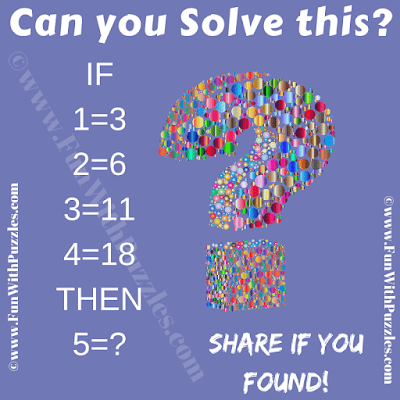This is the Maths Logical Reasoning Fun Puzzle to test your brain. In this Brain Test, you are shown some logical number equations. These logical equations follow a certain logical or mathematical pattern. Your challenge is to crack this logical hidden code and then find the value of the missing number in the last number equation. This is not a tough logic puzzle but it will test your IQ. Let's see if you can solve this mathematical logic puzzle correctly in your first attempt?Can you solve this Math Reasoning Fun Puzzle?
The answer to this "Mathematical Reasoning Fun Puzzle", can be viewed by clicking on the answer button.